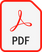# Mathematical Modeling in Digital Environment and its Implications on Teaching and Learning Extreme Values and Functions’ Monotonicity

9th International Scientific Conference Technics and Informatics in Education – TIE 2022 (2022) стр. 309-314

#### АУТОР(И): Tanja Sekulić, Valentina Kostić

Download Full Pdf#### САЖЕТАК:

In this paper, we present a way of introducing teaching and learning method based on the principles of mathematical modeling empowered by digital technologies. The role of mathematical modeling in educational practice is discussed as also the implications of the realization of mathematical modeling in a computer environment. In the example of teaching Extreme values and functions’ monotonicity, step by step procedure of making a mathematical model is explained – from starting preparation, to implementing GeoGebra as the software tool. The experiences of students and teachers during and after learning and teaching using mathematical modeling in the GeoGebra environment were supportive concerning further implications of this method.

#### КЉУЧНЕ РЕЧИ:

Mathematical modeling, GeoGebra, Extreme values, Functions’ monotonicity

#### ЛИТЕРАТУРА:

• Sekulic, T., Takaci, D., Strboja, M., & Kostic, V.(2020). Influence of Mathematical Modeling inGeoGebra Environment on LearningDerivative. The International Journal forTechnology in Mathematics Education, vol. 27,no. 2, 61-82.
doi: 10.1564/tme_v27.2.01
•  Galbraith, P. L., Stillman, G., & Brown, J. (2010). Turning Ideas into Modeling Problems. Modeling Students’ Mathematical Modeling Competencies, ICTMA 13, Section 4, New York: Springer, 133-144.
•  Valdés y Medina, E. G., & Medina Valdés, L. (2015). Dynamic Models as change Enablers in Educational Mathematics. Procedia – Social and Behavioral Sciences, Volume 176, 923-926.
•  Kostić, V., Stankov Jovanović, V., Sekulić, T. and Takači, Dj. (2016). Visualization of
problem solving related to the quantitative composition of solutions in the dynamic GeoGebra environment. Chemistry Education Research and Practice, vol. 17, no. 1, 120-138.
doi: 10.1039/c5rp00156k
•  Sekulić, T., Manigoda, G., Kostić, V. (2022). Teaching Trigonometry Online Using Geogebra Dynamical Materials. Sinteza 2022 – International Scientific Conference on Information Technology and Data Related Research, 121-128.
doi:10.15308/Sinteza-2022-121-128